# Magic square

(diff) ← Older revision | Latest revision (diff) | Newer revision → (diff)

A square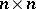array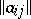composed of the integers from 1 up to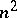and satisfying the following conditions: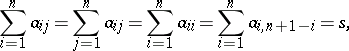(*)

where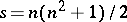. There are also more general magic squares, in whichis not required.

Any number,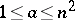, is uniquely characterized by a pair of residues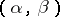(the digits to baseof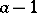), that is, by the points of the two-dimensional space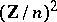over the ring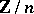of residues modulo. Since the coordinates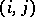of the cells of the square may also be regarded as the elements of, it follows that any distribution of the numbers from 1 up toin an arrayis given by a mappingthat is, by a pair of functions,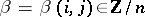, where. The problem is to investigate those pairs that give magic squares. In general this has been done (see ) only under the additional assumption of linearity ofand. It turns out, in particular, that magic squares with linearandexist for oddonly.

Already in the Middle Ages a number of algorithms for constructing magic squares of odd orderhad been found. Each such algorithm is characterized by six residues,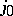,,,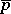,, and is described by the following rules: 1) the number 1 is put into the cell; and 2) ifwas put into, thenis put into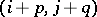if that cell is still empty or into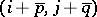ifis occupied.

The residues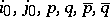are not arbitrary but must satisfy certain conditions to ensure not only that (*) holds, but also that the algorithm is feasible, that is, thatis empty whenis occupied. These conditions are easily found (see ). Moreover, it turns out that a magic square can be constructed by an algorithm of this type if and only if the functionsanddescribing the square are linear.

Many algorithms for constructing magic squares are known (resulting in squares with non-linearand), but there is no general theory for them (1989). Even the number of magic squares of orderis unknown (for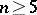; forthere is, up to obvious symmetries, only one magic square, whereas for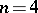there are 880 magic squares).

Magic squares having additional symmetry have also been investigated, again only in very special circumstances (for example, for; see ).

How to Cite This Entry:
Magic square. Encyclopedia of Mathematics. URL: http://encyclopediaofmath.org/index.php?title=Magic_square&oldid=13333
This article was adapted from an original article by M.M. Postnikov (originator), which appeared in Encyclopedia of Mathematics - ISBN 1402006098. See original article